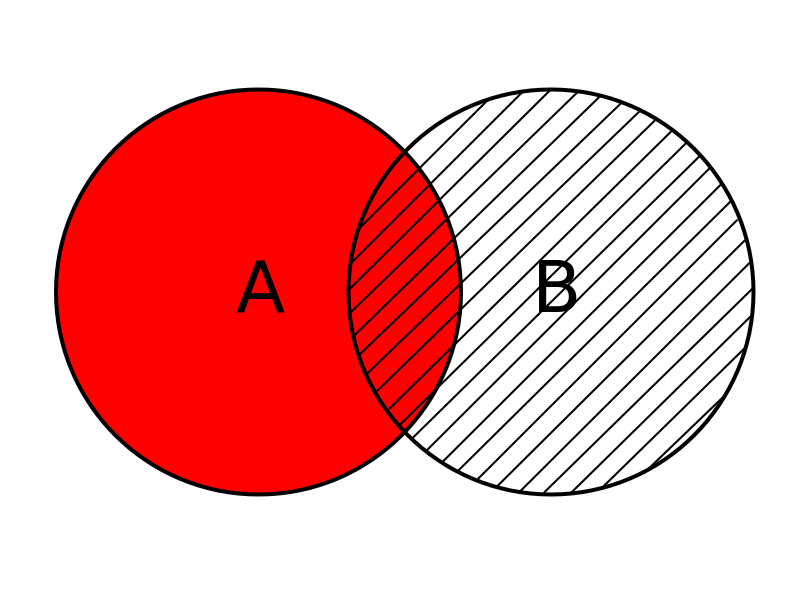# Fundamentals of probability part III

03/03/2020

## Instructions:

Use the left and right arrow keys to navigate the presentation forward and backward respectively. You can also use the arrows at the bottom right of the screen to navigate with a mouse.

FAIR USE ACT DISCLAIMER:
This site is for educational purposes only. This website may contain copyrighted material, the use of which has not been specifically authorized by the copyright holders. The material is made available on this website as a way to advance teaching, and copyright-protected materials are used to the extent necessary to make this class function in a distance learning environment. The Fair Use Copyright Disclaimer is under section 107 of the Copyright Act of 1976, allowance is made for “fair use” for purposes such as criticism, comment, news reporting, teaching, scholarship, education and research.

## Outline

• The following topics will be covered in this lecture:
• Review of conditional probability
• Review of the multiplication rule
• Review of independence
• Further notes on independence
• Further uses of conditional probability
• Bayes' Law

## Compound events

• We will often be concerned not with one event $$A$$ but some combination of some event $$A$$ and some event $$B$$.
• Compound event – formally we define a compound event as any event combining two or more simple events.
• There are two key operations joining events
1. “OR” – in mathematics we refer to “or” as a non-exclusive “or”.
• The meaning of this for “$$A$$ or $$B$$” is – event $$A$$ occurs, event $$B$$ occurs, or both events $$A$$ and $$B$$ occur.
• We will not consider the exclusive “or”, i.e. either event $$A$$ occurs, or event $$B$$ occurs, but not both.
2. “AND” – in mathematics we refer to “and” in an exclusive sense.
• The meaning of this for “$$A$$ and $$B$$” is – both event $$A$$ and event $$B$$ occurs.
• The operations “and” and “or” join events together in a way that we can compute the probability of the joint events.Courtesy of Bin im Garten CC via Wikimedia Commons

• Suppose we want to compute the probability of two events $$A$$ and $$B$$ joined by the compound operation “or”.
• We read the statement, $P(A \text{ or } B)$ as he probability of event:
• $$A$$ occuring,
• event $$B$$ occuring, or
• both $$A$$ and $$B$$ ocurring.
• Intuitively, we can express the probability in terms of all the ways $$A$$ can occur and all the ways $$B$$ can occur, if we don’t double count.
• Let all the ways that $$A$$ can occur be represented by the red circle to the left.
• Let all the ways that $$B$$ can occur be represented by the dashed circle to the left.
• If there is any overlap between events $$A$$ and $$B$$ so that they can occur simultaneously, $$P(A) +P(B)$$ counts the cases where $$A$$ and $$B$$ both occur twice.
• Therefore, the addition rule for compound events is given as, $P(A\text{ or }B) = P(A) + P(B) - P(A\text{ and }B)$

## Complementary eventsCourtesy of Bin im Garten CC via Wikimedia Commons

• A special case of the addition rule comes up when the events $$A$$ and $$B$$ are disjoint or mutually exclusive.
• When $$A$$ and $$B$$ are disjoint, this means that there is no overlap between these events and they will never occur simultaneously.
• In this case $$P(A \text{ and } B) = 0$$, so the addition rule becomes, \begin{align} P(A \text{ or } B) = P(A) + P(B) - P(A \text{ and } B) &= P(A) + P(B) \end{align}
• Recall the complement of $$A$$, denoted $$\overline{A}$$ is the event where $$A$$ does not occur.
• By definition, $$A$$ and $$\overline{A}$$ are disjoint because $$A$$ will not both occur and not occur simultaneously.
• However, complementary events make up all possible outcomes – $$A$$ will either occur or not occur, so that we are certain about the outcome of $$P(A \text{ or } \overline{A})$$.
• That is, we know by definition that $P(A \text{ or } \overline{A}) = 1$
• Using the above fact, along with the disjointness of $$A$$ and $$\overline{A}$$ with the addition rule, $1= P\left(A\text{ or }\overline{A}\right) = P(A) + P\left(\overline{A}\right).$ for any event $$A$$ and its complement $$\overline{A}$$.